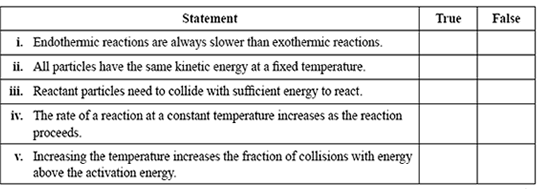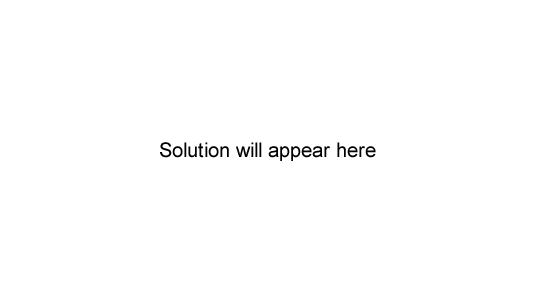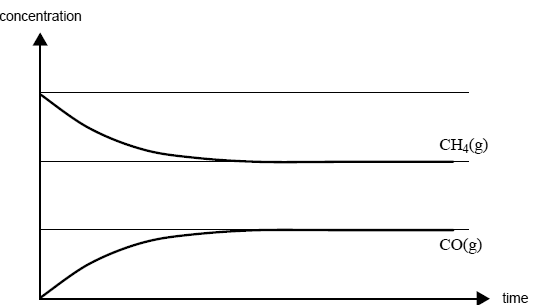Rates of reactions 2007 VCE The rates of chemical reactions may be explained using the collision theory model. Indicate whether the following statements about rates and the collision theory model are true or false by placing ticks in the appropriate boxes.SolutionCarbon monoxide and hydrogen can be produced from the reaction of methane with steam according to the equation CH4(g) + H2O(g) <=> CO(g) + 3H2(g); ΔH = +206 kJ mol-1 Some methane and steam are placed in a closed container and allowed to react at a fixed temperature. The following graph shows the change in concentration of methane and carbon monoxide as the reaction progresses.On the graph above, draw a line to show the change in concentration of hydrogen gas as the reaction progresses. Label this line. On the graph above, draw a line to show how the formation of carbon monoxide would differ over time in the presence of a catalyst. Label this line. Solution